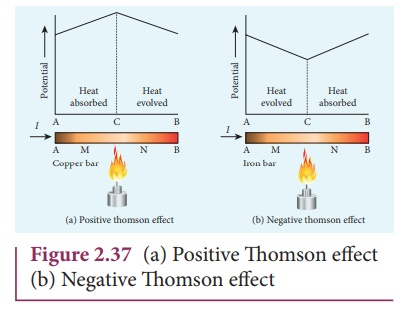Home | | Physics 12th Std | Thomson effect

# Thomson effect

Thomson showed that if two points in a conductor are at different temperatures, the density of electrons at these points will differ and as a result the potential difference is created between these points.

Conversion of temperature differences into electrical voltage and vice  versa is known as thermoelectric effect. A thermoelectric device generates voltage when there is a temperature difference on each side. If a voltage is applied, it generates a temperature difference.

Thomson effect

Thomson showed that if two points in a conductor are at different temperatures, the density of electrons at these points will differ and as a result the potential difference is created between these points. Thomson effect is also reversible.If current is passed through a copper bar AB which is heated at the middle point C, the point C will be at higher potential. This indicates that the heat is absorbed along AC and evolved along CB of the conductor as shown in Figure 2.37(a). Thus heat is transferred due to the current flow in the direction of the current. It is called positive Thomson effect. Similar effect is observed in metals like silver, zinc, and cadmium.

When the copper bar is replaced by an iron bar, heat is evolved along CA and absorbed along BC. Thus heat is transferred due to the current flow in the direction opposite to the direction of current. It is called negative Thomson effect as shown in the Figure 2.37(b). Similar effect is observed in metals like platinum, nickel, cobalt, and mercury.

Tags : Thermoelectric Effect , 12th Physics : Current Electricity
Study Material, Lecturing Notes, Assignment, Reference, Wiki description explanation, brief detail
12th Physics : Current Electricity : Thomson effect | Thermoelectric Effect